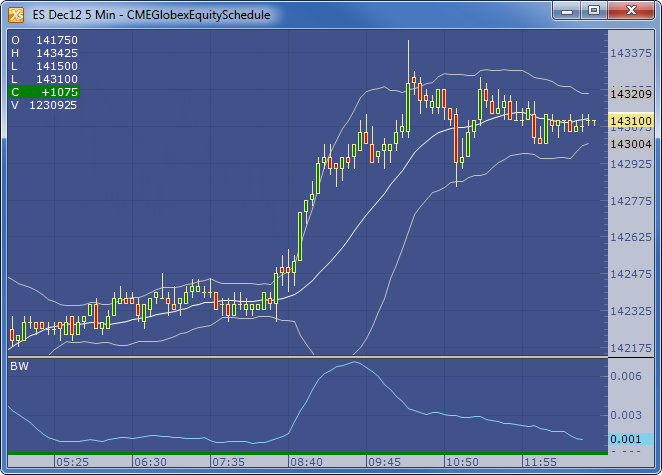← Back to X_TRADER® Help Library

### X_STUDY® Documentation

#### Band Width (BW)

##### Description

The width of the bands is based on the standard deviation of the closing prices from a moving average of price. You can apply this study to any band study, such as Keltner channel.

##### Formula

Simplified:

Middle Band = n-period moving average

Upper Band = Middle Band + ( y * n-period standard deviation)

Lower Band = Middle Band - ( y * n-period standard deviation)

Where:

• n = number of periods
• y = factor to apply to the standard deviation value, (typical default for y = 2)

Detailed:

1. Calculate the moving average. The formula is:
2.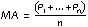• Pn is the price you pay for the nth interval
• n is the number of periods you select
3. Subtract the moving average from each of the individual data points used in the moving average calculation. This gives you a list of deviations from the average. Square each deviation and add them all together. Divide this sum by the number of periods you selected.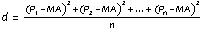4. Take the square root of d. This gives you the standard deviation.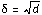5. Compute the bands by using the following formulas: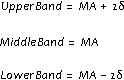##### Example